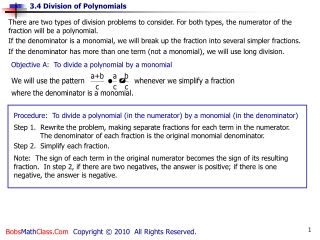DownloadDownload PresentationProcedure: To divide a polynomial (in the numerator) by a monomial (in the denominator)

# Procedure: To divide a polynomial (in the numerator) by a monomial (in the denominator)

Télécharger la présentation## Procedure: To divide a polynomial (in the numerator) by a monomial (in the denominator)

- - - - - - - - - - - - - - - - - - - - - - - - - - - E N D - - - - - - - - - - - - - - - - - - - - - - - - - - -
##### Presentation Transcript

1. There are two types of division problems to consider. For both types, the numerator of the fraction will be a polynomial. If the denominator is a monomial, we will break up the fraction into several simpler fractions. If the denominator has more than one term (not a monomial), we will use long division. Objective A: To divide a polynomial by a monomial Procedure: To divide a polynomial (in the numerator) by a monomial (in the denominator) Note: The sign of each term in the original numerator becomes the sign of its resulting fraction. In step 2, if there are two negatives, the answer is positive; if there is one negative, the answer is negative. Step 1. Rewrite the problem, making separate fractions for each term in the numerator. The denominator of each fraction is the original monomial denominator. Step 2. Simplify each fraction.

2. Your Turn Problem #1 1. Separate into three fractions, one for each term in the numerator. 2. Simplify each fraction.

3. Your Turn Problem #2 1. Separate into three fractions, one for each term in the numerator. 2. Simplify each fraction.

4. Objective B: To divide a polynomial by a polynomial Procedure: To divide a polynomial (in the numerator) by a polynomial in the denominator Step 2. Divide the first term of the dividend by the first term of the divisor. Write the answer in the quotient. Step 3. Multiply answer from Step 2 by the divisor. Write answer directly under the first term of the dividend. Step 4. Subtract (change signs) answer found in Step 3 from the dividend. Add the columns and write the answer directly below, under a horizontal bar. Step 5. Bring down the next term of the dividend under the horizontal bar, next to the difference found in Step 4. Step 6. Repeat steps 2 to 5 using the polynomial under the horizontal bar as the new dividend. Step 7. If no terms are left to bring down, then the polynomial remaining under the horizontal bar (if any) is the remainder. Write the remainder in fractional form.

5. Your Turn Problem #3 1. Rewrite in long division format. 2. Divide first term of dividend by first term of divisor. Write in quotient. 3. Multiply 2x by the divisor. Write below dividend. 4. Write a line below and change the signs. 5. Add the columns and bring down next term. 6. Repeat the steps. Divide first term (from step 5) by the first term of the divisor. Write in quotient. 7. Multiply the divisor by 1. 8. Write a line below and change the signs. 9. Add columns. Note: To check the answer, multiply the divisor and the quotient which should equal the dividend. i.e. Multiply (x+2)(2x+1)

6. Your Turn Problem #4 1. Rewrite in long division format. 2. Divide first term of dividend by first term of divisor. Write in quotient. 3. Multiply x2 by the divisor. Write below dividend. 4. Write a line below and change the signs. 5. Add the columns and bring down next term. 6. Repeat the steps. Divide first term (from step 5) by the first term of the divisor. Write in quotient. 7. Multiply the divisor by –5x. 8. Write a line below and change the signs. 9. Add columns, bring down next term. 10. Divide first term by the divisor; write in quotient. 11. Multiply 4 by divisor. 12. Write a line below and change the signs. To check: multiply divisor and quotient, then add remainder. This will give the dividend. 13. Add columns, this is the remainder.

7. 1. The polynomials must be in descending order. 2. If the dividend has a gap in the exponent sequence, insert the variable(s) with a coefficient of 0. Next Slide Note: There are two conditions which are to be followed before the steps for long division can be performed.

8. Your Turn Problem #5 1. Rewrite in long division format. 2. Divide first term of dividend by first term of divisor. Write in quotient. 3. Multiply x2 by the divisor. Write below dividend. 4. Write a line below and change the signs. 5. Add the columns and bring down next term. 6. Repeat the steps. Divide first term (from step 5) by the first term of the divisor. Write in quotient. 7. Multiply the divisor by 2x. 8. Write a line below and change the signs. 9. Add columns, bring down next term. 10. Divide first term by the divisor; write in quotient. 11. Multiply 4 by divisor. 12. Write a line below and change the signs. 13. Add columns. This is the remainder. The End. B.R. 12-28-06